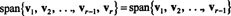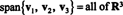## Linear Combinations and Span

Let v 1, v 2,…, v r be vectors in R n . A linear combination of these vectors is any expression of the formwhere the coefficients k 1, k 2,…, k r are scalars.

Example 1: The vector v = (−7, −6) is a linear combination of the vectors v1 = (−2, 3) and v2 = (1, 4), since v = 2 v1 − 3 v2. The zero vector is also a linear combination of v1 and v2, since 0 = 0 v1 + 0 v2. In fact, it is easy to see that the zero vector in R n is always a linear combination of any collection of vectors v1, v2,…, vr from Rn.

The set of all linear combinations of a collection of vectors v1, v2,…, vr from Rn is called the span of { v1, v2,…, vr }. This set, denoted span { v1, v2,…, vr}, is always a subspace of R n , since it is clearly closed under addition and scalar multiplication (because it contains all linear combinations of v1, v2,…, v r). If V = span { v 1, v 2,…, v r }, then V is said to be spanned by v 1, v 2,…, v r .

Example 2: The span of the set {(2, 5, 3), (1, 1, 1)} is the subspace of R 3 consisting of all linear combinations of the vectors v 1 = (2, 5, 3) and v 2 = (1, 1, 1). This defines a plane in R 3. Since a normal vector to this plane in n = v 1 x v 2 = (2, 1, −3), the equation of this plane has the form 2 x + y − 3 z = d for some constant d. Since the plane must contain the origin—it's a subspace— d must be 0. This is the plane in Example 7.

Example 3: The subspace of R 2 spanned by the vectors i = (1, 0) and j = (0, 1) is all of R 2, because every vector in R 2 can be written as a linear combination of i and j:Let v 1, v 2,…, v r−1 , v r be vectors in R n . If v r is a linear combination of v 1, v 2,…, v r−1 , thenThat is, if any one of the vectors in a given collection is a linear combination of the others, then it can be discarded without affecting the span. Therefore, to arrive at the most “efficient” spanning set, seek out and eliminate any vectors that depend on (that is, can be written as a linear combination of) the others.

Example 4: Let v 1 = (2, 5, 3), v 2 = (1, 1, 1), and v 3 = (3, 15, 7). Since v 3 = 4 v 1 − 5 v 2That is, because v 3 is a linear combination of v 1 and v 2, it can be eliminated from the collection without affecting the span. Geometrically, the vector (3, 15, 7) lies in the plane spanned by v 1 and v 2 (see Example 7 above), so adding multiples of v 3 to linear combinations of v 1 and v 2 would yield no vectors off this plane. Note that v 1 is a linear combination of v 2 and v 3 (since v 1 = 5/4 v 2 + 1/4 v 3), and v 2 is a linear combination of v 1 and v 3 (since v 2 = 4/5 v 1 − 1/5 v 3). Therefore, any one of these vectors can be discarded without affecting the span:Example 5: Let v 1 = (2, 5, 3), v 2 = (1, 1, 1), and v 3 = (4, −2, 0). Because there exist no constants k 1 and k 2 such that v 3 = k 1 v 1 + k 2 v 2, v 3 is not a linear combination of v 1 and v 2. Therefore, v 3 does not lie in the plane spanned by v 1 and v 2, as shown in Figure :Figure 1

Consequently, the span of v 1, v 2, and v 3 contains vectors not in the span of v 1 and v 2 alone. In fact,